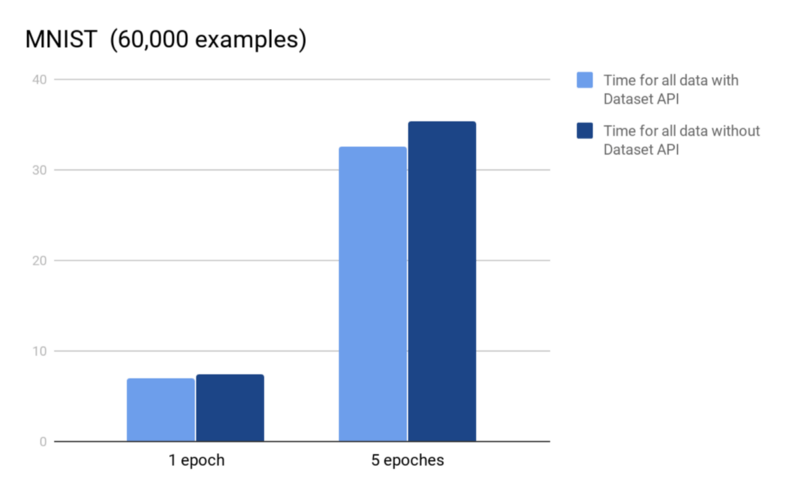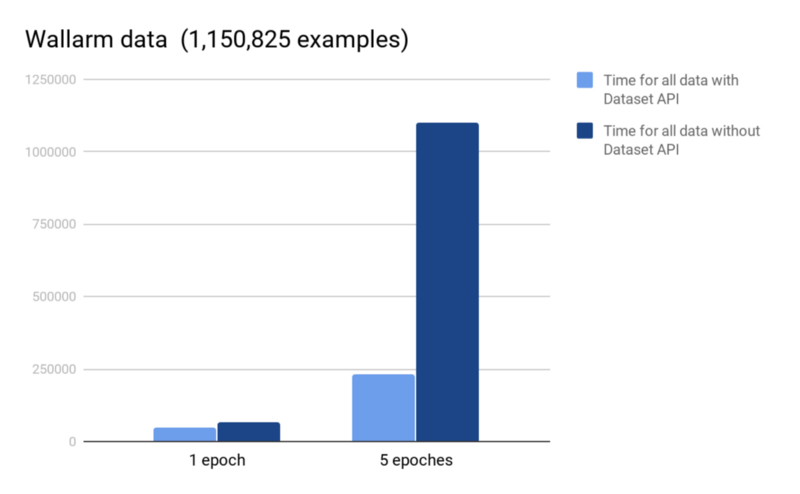#### by M.Salnikov, Wallarm Research

Wallarm AI engine is the heart of our security solution. Two key parameters of our AI engine efficiency are how fast neural networks can be train to reflect the updated training sets and how much compute power need to be dedicated to the training on the on-going basis.

Many of our machine learning algorithms are written on top of TensorFlow, an open-source dataflow software library originally release by Google.

Our average CPU load for the AI engine today is as high as 80% so we are always looking for ways to speed things up in software. Our latest find is Dataset API. Dataset is a mid-level TensorFlow APIs which makes working with data faster and more convenient..

In this blog, we will measure just how much faster model training can be with Dataset, compared to the you use of `feed_dict`.

For starters, let’s prepare data that will be used to train the model. Dataset can usually be stored in numpy’s arrays regardless of kind of data they are.. That’s why we prepare all our dataset without TensorFlow and store it in .npz format similar to this:

```train_x, train_y = preprocessing_as_np(train_data)
test_x, test_y = preprocessing_as_np(test_data)

np.savez(os.path.join(dataset_path, "train"),
x=train_x,
y=train_y)

np.savez(os.path.join(dataset_path, "test"),
x=test_x,
y=test_y)```

https://github.com/wallarm/researches/blob/a719923f6a2da461deea0e01622d11cbfc8b057b/tf_ds_api/storing_in_npz_format.py#L1-L10

This step helps us avoid unnecessary data processing load on CPU and memory during model training.

Now we are ready to train the model. First, let’s load preprocessed data from disk:

```with np.load(os.path.join(dataset_path, "train.npz")) as data:
train_x=data['x']
train_y=data['y']

train_x=data['x']
train_y=data['y']```

Next the data will be converted from numphy arrays into TensorFlow tensors (tf.data.Dataset.from_tensor_slices method is used for that) and loaded into TensorFlow. Dataset.from_tensor_slices method takes placeholders with the same size of the 0th dimension element and returns dataset object.

Once the dataset is in TF, you can process it, for example, you can use .map(f) function which can process the data. But we already preprocess our dataset and all we need to do is apply batching and, maybe, shuffling. Fortunately, Dataset API already has needed functions. They are .batch and .shuffle. Ok, if we shuffle our dataset how can we use it for production? It’s easy, we simply make another dataset without data been shuffled.

```x_ph = tf.placeholder(tf.int32, [None]+
list(train_x.shape[1:]), name="x")
y_ph = tf.placeholder(tf.int32, [None]+
list(train_y.shape[1:]), name="y")

train_dataset = tf.data.Dataset.from_tensor_slices
((x_ph, y_ph)).shuffle(buffer_size=10000).batch(BATCH_SIZE)
valid_dataset = tf.data.Dataset.from_tensor_slices
((x_ph, y_ph)).batch(BATCH_SIZE)```

https://github.com/wallarm/researches/blob/a719923f6a2da461deea0e01622d11cbfc8b057b/tf_ds_api/datasets.py#L1-L5

Dataset API has other good methods for preprocessing data. There is a comprehensive list of methods in the. official docs.

Next we should extract data from dataset object step by step for each of the training epochs, tf.data.Iterator is tailor-made for it. TF currently supported four type of iterators:

1. One-shot — is the simplest iterator. The usage is very simple, but only a single dataset is supported. Initializable — requires that iterator.initializer is run before it can be used This method is not quite as convenient as one-shot, but we are getting a method that is better suited for working with datasets.
2. Reinitializable — IMHO, it’s the most useful type of an iterator. As the name implies, this iterator can be initialized withdifferent datasets. In this blog post, we use this type of an iterator.
3. Feedable — is used together with placeholders to choose what iterator to use in each call.

Reinitializeble iterator is very useful, all we need to do to start the work is to create an iterator and initializers for it. iterator.get_next() yields the next elements of our dataset when executed.

```iterator = tf.data.Iterator.from_structure(train_dataset.output_types,
train_dataset.output_shapes)
next_elements = iterator.get_next()

training_init_op = iterator.make_initializer(train_dataset, name="training_init_op")
validation_init_op = iterator.make_initializer(valid_dataset, name="validation_init_op")

x, y = next_elements```

https://github.com/wallarm/researches/blob/a719923f6a2da461deea0e01622d11cbfc8b057b/tf_ds_api/iterator.py#L1-L8

### Experiment

To demonstrate the viability of using Dataset API let’s use proposed approach for MNIST dataset and for our corporate data . First, we prepared data and after that, we processed 1 and 5 epochs with Dataset API and without. Model for this MNIST example can be found on github:

```class Model(object):
def __init__(self, x, y,
hidden_layer_0 = tf.layers.dense(x, 1024, activation=tf.nn.relu)
hidden_layer_1 = tf.layers.dense(hidden_layer_0, 784, activation=tf.nn.relu)
hidden_layer_2 = tf.layers.dense(hidden_layer_1, 512, activation=tf.nn.relu)
logits = tf.layers.dense(hidden_layer_2, 10, activation=tf.nn.softmax)
self._loss = tf.losses.softmax_cross_entropy(tf.one_hot(y, 10), logits)
self._global_step = tf.Variable(0, trainable=False, name="global_step")

self._train_op = tf.contrib.layers.optimize_loss(loss=self._loss,
optimizer=optimizer,
global_step=self._global_step,
learning_rate=learning_rate,
name="train_op",
summaries=['loss'])

self._summaries = tf.summary.merge_all()

if not os.path.exists(run_dir):
os.mkdir(run_dir)
if not os.path.exists(os.path.join(run_dir, "checkpoints")):
os.mkdir(os.path.join(run_dir, "checkpoints"))
self._run_dir = run_dir
self._saver = tf.train.Saver(max_to_keep=1)```

https://github.com/wallarm/researches/blob/a719923f6a2da461deea0e01622d11cbfc8b057b/tf_ds_api/model.py#L1-L25

Below are the results we obtained on a machine with one Nvidia GTX 1080 and TF 1.8.0.All code of this experiment is available on GitHub [Link].

MNIST is a very small dataset and profit of Dataset API isn’t representative. By contrast, the results on a real-life dataset are much more impressive.Thus Dataset API is very good for increasing your training speed. With no source code changes, just some modifications in the stack, you can save 20–30% off the training time.